# Inversion: 1D Magnetotelluric Problem¶

In the previous notebooks, we explored how to discretize the 1D MT problem, how to design an appropriate mesh, and explored the an example of non-uniquness. In this notebook, we will put those concepts to use and set up a 1D inversion.

The aim of this notebook is to highlight the elements we use to set up and solve the inverse problem, in the notebook that follows this, we will dive futher into the impact of various parameter choices (eg. the trade-off parameter $\beta$, the stopping criteria, noise model and regulariztion parameters).

## Inversion as Optimization¶

We will use deterministic approach and pose the inverse problem as an optimization problem of the form

$$\min_{\mathbf{m}} \phi(\mathbf{m}) = \phi_d(\mathbf{m}) + \beta\phi_m(\mathbf{m})$$

where:

• $\mathbf{m}$ is our model - the vector of numbers that describes our earth model. It is what we are solving for in the inversion
• $\phi_d(\mathbf{m})$ is the data misfit
• $\phi_m(\mathbf{m})$ is the regularization
• $\beta$ is a trade-off parameter

### Data Misfit¶

The data misfit, $\phi_d(\mathbf{m})$, is often taken to be a weighted $\ell_2$-norm, where the weights capture the noise model (eg. we want to assign higher weights and do a good job fitting data that we are confident are less noisy, and assign less weight / influence to data that are noisy). The $\ell_2$ norm is the correct norm to choose when noise is Gaussian (or approximately Gaussian, or if you have no additional information and assume it is Gaussian). An $\ell_2$ data misfit is captured mathematically by

$$\phi_d(\mathbf{m}) = \frac{1}{2}\|\mathbf{W_d} (\mathcal{F}(\mathbf{m}) - \mathbf{d}^{\text{obs}})\|^2$$

where

• $\mathbf{W_d} = \text{diag}(1/\boldsymbol{\epsilon})$ is a diagonal matrix with diagonal entries $1/\epsilon_i$, where $\epsilon_i$ is an estimated standard deviation of the $i$th datum.
• $\mathcal{F}$ is the forward modelling operator that simulates the 1D MT response given a model
• $\mathbf{m}$ is the model: the set of parameters which describe the physical properties of the layered earth
• $\mathbf{d}^{\text{obs}}$ is the observed data

(The factor of $1/2$ and the choice of using the squared-norm is a matter of convienence. We will be using gradient based optimzation methods, so it is easier to take derivatives of a norm squared than a norm.)

### Regularization¶

The inverse problem is an ill posed problem. There are multiple (actually infinitely many!) models that can fit the data. There are a couple ways to observe this:

• Mathematically: If we start by thinking about a linear problem $\mathbf{G}\mathbf{m} = \mathbf{d}$, the matrix $\mathbf{G}$ is wide, so it is not directly invertible (eg. see Matt Hall's Linear Inversion Tutorial). Here, we are dealing with a non-linear system of equations, but the principle is the same.

• An Example: In the Forward Modelling and Nonuniqueness notebook, we used forward modelling to demonstrate non-uniquness with the conductivity-thickness product of a single, conductive layer, and this is a very simple model compared to most geologic settings!

Thus, to choose from the infinitely many solutions and arrive at a sensible one, we employ a regularization: $\phi_m$. Tikhonov regularization, which again employs $\ell_2$-norms, is a standard choice (It has a few nice features: it is convex and easy to differentiate). It takes the form: $$\phi_m(\mathbf{m}) = \frac{1}{2}\big(\alpha_s\|\mathbf{W_s} (\mathbf{m} - \mathbf{m}_{\text{ref}})\|^2 + \alpha_z\|\mathbf{W_z} (\mathbf{m})\|^2 \big)$$

The first term is often referred to as the "smallness" as it measures the "size" of the model (in the $\ell_2$ sense). The matrix $\mathbf{W_s}$ is generally taken to be a diagonal matrix that may contain information about the length scales of the model or be used to weight the relative importance of various parameters in the model. The scalar $\alpha_s$ weights the relative importance of this term in the regularization. Notice that we include a reference model ($\mathbf{m}_{\text{ref}}$. Often this is defined as a constant value, but if more information is known about the background, that can be used to construct a reference model. Note that saying "I am not going to use a reference model" means that you are actually using $\mathbf{m}_{\text{ref}} = 0$, this is important to realize... in the inversion we demonstrate here, our model will be $\mathbf{m} = \text{log}(\sigma)$. If we set $\mathbf{m}_{\text{ref}} = 0$, then we are favoring models close to 1 S/m - which is quite conductive!

The second term is often referred to as the "smoothness". The matrix $\mathbf{W_z}$ approximate the derivative of the model with respect to depth, and is hense a measure of how "smooth" the model is. The term $\alpha_z$ weights its relative importance in the regularization.

## In this notebook...¶

Although we pose the inverse problem as an optimization problem, we aren't necessarily going to solve to a true minimum. There are always uncertainties with the data, so there is no point trying to drive $\phi_d$ to its true minimium - we would end up introducing structures in the model to fit the data (we will explore this here). So another choice that has to be made when setting up an inversion is where to stop.

In this notebook, we will walk through how to set up an inversion and discuss one of the knobs:

• The $\beta$ knob: How well should we fit the data verses the regularization? Which $\beta$ value provides us the right balance? eg. When do we achieve the target misfit $\phi_d^*$?
• The $\alpha$ knob: Tuning the regularization ($\alpha_s$, $\alpha_z$): how smooth or small (in the $\ell_2$-sense) do we want our model to be?

We will also point out other parameters you can explore the impact of, including,

• starting model $\mathbf{m}_0$
• reference model $\mathbf{m}_{\text{ref}}$
• designing a $\beta$-cooling schedule

In the next notebook we will dive further into the $\alpha$ knob.

A Note

We will employ second-order optimization methods, meaning we have to be able to both simulate data and compute derivatives. We will take those steps for granted in this notebook, but if you are curious to look under the hood and see how sensitivities are computed for this problem, have a look at the Sensitivities Notebook.

In :
from scipy.constants import mu_0
import numpy as np
import matplotlib.pyplot as plt

from SimPEG import (
Mesh, Maps, SolverLU, DataMisfit, Regularization,
Optimization, InvProblem, Inversion, Directives, Utils
)

try:
from pymatsolver import PardisoSolver as Solver
except importError:
from SimPEG import SolverLU as Solver

from MT1D import MT1DProblem, MT1DSurvey, MT1DSrc, ZxyRx, Survey, AppResPhaRx
%matplotlib inline


## Forward Simulation¶

### Parmeters¶

Now, we define our model parameters and survey setup. This includes defining

• frequencies we are sampling
• type of dat we are measuring (impedance or apparent resistivity and phase)

Similar to the model shown in 1_MT1D_NumericalSetup.ipynb and 2_MT1D_ForwardModellingAndNonuniqueness. We will consider a model which consists of 5 units:

In :
layer_tops = np.r_[0., -600., -1991., -5786., -9786.]  # in m
rho_layers = np.r_[250., 25, 100., 10., 25.]

In :
rxloc = np.r_[0.]
frequency = np.logspace(-3, 2, 25)


### Survey¶

Next, we set up a survey object. Here we are following the SimPEG approach and define

• a receiver object: given fields found from a simulation, it has the machinery to compute data. An impedance receiver ZxyRx calculates the ratio of $E_x$ and $H_y$ from calculated electric and magnetic fields (both real and imaginary components)
$$Z_{xy} = - \frac{E_x}{H_y}$$
• a source object: this provides the right-hand-side for our MT problem. This is a plane wave source with at the specified frequency.

These are combined in a survey.

In :
# Create a receiver object
rx = ZxyRx(
rxloc, # location of the receiver
component="both", # measure both the real and imaginary components of the impedance (alternatively "real" / "imag")
frequency=frequency
)
# create a plane wave source
src = MT1DSrc([rx])

# define a survey
survey = MT1DSurvey([src])


### Mesh¶

In the Forward Modelling and Nonuniqueness notebook, we discussed how to design a mesh that extends sufficiently far and has fine enough cells near the surface to accurately simulate the MT response across the frequency range of interest. We have wrapped up that knowledge in the utility function setMesh.

Since most of the geologic units we are considering are on the order of $100 \Omega m$, we will use a conductivity value of $10^{-2}$ S/m for creating the mesh.

In :
max_depth_core = 15000.
mesh = survey.setMesh(
sigma=0.01, # approximate conductivity of the background
max_depth_core=max_depth_core,  # extent of the core region of the mesh
ncell_per_skind=10,  # number of cells per the smallest skin depth
n_skind=2,  # number of skin depths that the mesh should extend to ensure the lowest-frequency fields have decayed
core_meshType = "log",   # cell spacings in the core region of the mesh ("linear" or "log")
max_hz_core=1000.  # If using a logarithmic core mesh, what is the maximum cell size?
)

>> Smallest cell size = 50 m
>> Padding distance = 316227 m
>> # of padding cells 17
>> # of core cells cells 47

In :
# plot the mesh
fig, ax = plt.subplots(1,1, figsize=(8, 3))
mesh.plotGrid(centers=True, faces=True, ax=ax)
ax.legend(["centers", "faces"])
ax.invert_xaxis()  # so that the surface is on our left hand side
ax.set_xlabel('z (m)')
ax.grid(which="both", linewidth=0.5)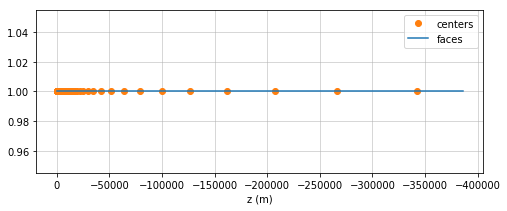### Problem¶

In the first notebook, we set up the machinery to solve the 1D MT problem. Here, we will use that functionality. Please see Sensitivities Notebook for further discussion on how MT1DProblems is set up.

In :
prob = MT1DProblem(
mesh,  # The mesh contains the geometry, grids, etc necessary for constructing the discrete PDE system
sigmaMap=Maps.ExpMap(mesh),  # in the inversion, we want to invert for log-conductivity (enforces postivity, electrical conductivity tends to vary logarithmically)
verbose=False,  # print information as we are setting up and solving
Solver=Solver  # solver to employ for solving Ax = b
)

# tell the problem and survey about each other so we can construct our matrix system
# and right hand-side
prob.pair(survey)


### Put the model on the mesh¶

Physical properties are defined at cell centers, so there should be a sigma value for every cell center. Above, we defined our model as resisvitities (in units of $\Omega m$), but for the inversion, we want to work in conductivities (in units of S/m), so we take the reciprocal ($\sigma = 1/\rho$)

In :
# start with nans so we can do a check to make sure all
# layer conductivities have been properly assigned
rho = np.ones(mesh.nC) * np.nan

# loop over each layer in the model and assign to mesh
for layer_top, rho_layer in zip(layer_tops, rho_layers):
inds = mesh.vectorCCx < layer_top
rho[inds] = rho_layer

sigma = 1./rho

In :
# plot the model
fig, ax = plt.subplots(1, 1, figsize=(8, 3))

# trickery to plot from node to node rather than at cell centers
z = np.repeat(mesh.vectorCCx[1:], 2, axis=0)
z = np.r_[mesh.vectorCCx, z, mesh.vectorCCx[-1]]
sigma_plt = np.repeat(sigma, 2, axis=0)

ax.loglog(-z, sigma_plt, lw=2)
ax.set_ylabel("Conductivity (S/m)", fontsize=13)
ax.set_xlabel("Depth (m)", fontsize=13)
ax.grid(True, which='both', linewidth=0.4)
ax.set_ylim(2e-3, 2e-1)
ax.set_xlim(0, max_depth_core*2.)

/anaconda3/lib/python3.6/site-packages/matplotlib/axes/_base.py:2966: UserWarning: Attempted to set non-positive xlimits for log-scale axis; invalid limits will be ignored.
'Attempted to set non-positive xlimits for log-scale axis; '

Out:
(25.00000000023283, 30000.0)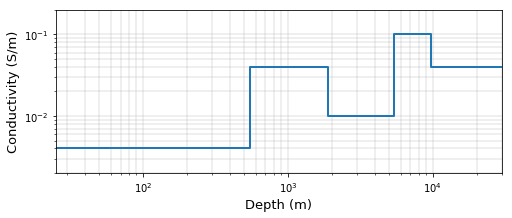## Simulate 'Observed' Data¶

Based on the conductivity structure plotted above, we will create synthetic data. These data will later be used in the inversion.

In the inversion, the "model" that we will invert for is in $\log(\sigma)$. There are a couple of reasons for this: the electrical conductivity of earth materials can vary over several orders of magnitude, and electrical conductivity is always positive, so inverting for $\log(\sigma)$ enforces that.

In SimPEG, the method dpred of the survey class solves the PDE and computes the data required by the receivers.

In :
mtrue = np.log(sigma)  # since our "model" is log conductivity, we take the log
dtrue = survey.dpred(mtrue)  # these are clean data (no noise yet.)


Add noise to generate "observed" data

In :
np.random.seed(1)  # set a seed to the results are reproducable
std = 0.1  # standard deviation of the noise (10%)

uncert = std * np.abs(dtrue)
noise = uncert * np.random.randn(survey.nFreq*2)
survey.dobs = dtrue + noise


Although we will work with real and impaginary impedance values as our data in the inversion, it is a bit more intuitive to plot the data in terms of apparent resistivity (which hase units of resistivity, $\Omega m$) and phase

$$\rho_a = \frac{1}{\mu_0\omega} \big|Z_{xy}\big|^2$$$$\phi = \tan^{-1}\left(\frac{\text{Im}(Z_{xy})}{\text{Re}(Z_{xy})}\right)$$
In :
def omega(frequency):
"""
angular frequency
"""
return 2*np.pi*frequency

def appres_phase_from_data(data, frequency):
"""
Compute apparent resistivity and phase given impedances (real and imaginary components)
and the frequency.
"""

# data are arranged (Zxy_real, Zxy_imag) for each frequency
Zxy_real = data.reshape((survey.nFreq, 2))[:,0]
Zxy_imag = data.reshape((survey.nFreq, 2))[:,1]
Zxy = Zxy_real+1j*Zxy_imag

# compute apparent resistivity and phase from complex impedance
app_res = abs(Zxy)**2 / (mu_0*omega(frequency))

return app_res, phase

In :
# fetch the apparent resistivity and phase for the clean (true)
# and noisy (obs) data
app_res_true, phase_true = appres_phase_from_data(dtrue, frequency)
app_res_obs, phase_obs = appres_phase_from_data(survey.dobs, frequency)

In :
fig, ax = plt.subplots(2, 1, figsize=(8,6))

# apparent resistivity
ax.loglog(frequency, app_res_true, '-k', lw=1, label="clean")
ax.loglog(frequency, app_res_obs, '-o', lw=1, label="noisy")
ax.set_ylabel("$\\rho_a \ (\Omega m)$", fontsize = 14)
ax.set_ylim([10, 3e2])

# phase
ax.semilogx(frequency, phase_true, '-k', lw=1, label="clean")
ax.semilogx(frequency, phase_obs, '-o', lw=1, label="noisy")
ax.set_ylabel("Phase (degree)")
ax.set_ylim([30, 80])

for a in ax:
a.grid(True, which='both', linewidth=0.4)
a.legend()
a.set_xlim(frequency.max(), frequency.min())
a.set_xlabel("Frequency (Hz)")

plt.tight_layout()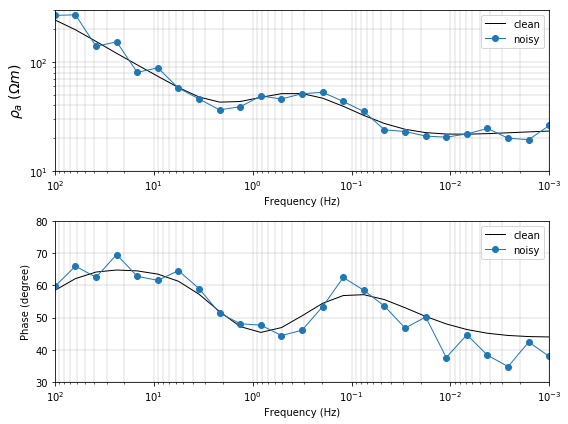## Inverse Problem: Machinery¶

Our setup of the inversion follows the SimPEG framework.The "inversion implementation" consists of 8 modules, 3 of which we have already been working with. This is a very brief overview. For more details, see the SimPEG docs

• Mesh: mesh geometry and differential operators
• Problem: physics engine. contains the machinery to construct and solve the PDE
• Survey: sources and receivers
• Data Misfit: measure of how "far" the predicted data are from the observed data
• Regularization: Regularization on the model. Here we use Tikhonov regularization
• InvProb: statement of the inverse problem, brings together the data misfit and regularization to define an objective function that we minimize in the inversion
• Optimization: algorithm we will use to perform the optimization in the inversion, here, we choose a gradient based approach
• Inversion: Bring everything together to run the inversion. This includes directives which are instructions on updates that should be made during the course of the inversion (eg. cooling beta) and stopping criteria (eg. target misfit)
In :
def run_MT1Dinv(
prob,  # 1D MT problem
survey,  # 1D MT survey with sources and receivers
m0,  # starting model
mref=None,  # reference model
alpha_s=1.,  # smallness weight
alpha_z=1.,  # smoothness weight
use_betaest=False,  # estimate an initial beta based on the the data misfit and regularization terms
beta0_ratio=None , # if we are estimating beta, how much should we favor the regularization?
coolingFactor=1.5,  # cooling factor
coolingRate=1,  # cool beta after this many iterations
use_target=True,  # stop the inversion at the target misfit?

):
# if the starting model is not defined, use the reference model
if mref is None:
mref = m0

# Data misfit
dmisfit = DataMisfit.l2_DataMisfit(survey)
dmisfit.W = 1./uncert

# Regularization
reg = Regularization.Simple(
prob.mesh, alpha_s=alpha_s, alpha_x=alpha_z, mref=mref
) # since we are in 1D, we work with the first dimension

# Optimization
opt = Optimization.InexactGaussNewton(maxIter=35, LSshorten=0.05)

# Statement of the inverse problem
invProb = InvProblem.BaseInvProblem(dmisfit, reg, opt)

# Inversion Directives
beta = Directives.BetaSchedule(
coolingFactor=coolingFactor, coolingRate=coolingRate
)
invProb.beta = beta0
invProgress = Directives.SaveOutputEveryIteration(save_txt=False)
target = Directives.TargetMisfit()
directives = [beta, invProgress]

if use_target:
directives.append(target)
if use_betaest:
if beta0_ratio is None:
beta0_ratio = 1.
betaest = Directives.BetaEstimate_ByEig(beta0_ratio=beta0_ratio)
directives.append(betaest)

# assemble in an inversion
inv = Inversion.BaseInversion(invProb, directiveList=directives)
prob.counter = opt.counter = Utils.Counter()
opt.remember('xc')

# run the inversion
mopt = inv.run(m0)
xc = opt.recall("xc")
return xc, invProgress, reg


## Inverse Problem: Choices¶

### Target Misfit and the ideal $\beta$¶

If the noise is Gaussian, then the sum of squares (our data misfit) is a Chi-squared distribution, which has an expected value of $N_\text{data}$ (in our case, we divide this by two to match our definition of $\phi_d$). Thus, the ideal choice of $\beta$ is one that gives us $$\phi_d^* \approx \frac{1}{2} N_\text{data}$$

You can choose to stop the inversion once it achieves the target misfit (use_target=True). For this example, we want to also demonstrate what happens when you over-fit the data, so we will not stop it at the target misfit, but run the inversion until it hits the maximum number of iterations.

#### A (longish) note: $\beta$ cooling¶

In this example, we will run an inversion where we cool (reduce the value of) beta every iteration. In practice, beta cooling strategy is often applied in non-linear inversions. The data misfit contribution objective function is non-convex, meaning that it has more than one local minimum. The Tikhonov regularization is convex. Having a large beta ensures that at least locally, the function is convex, so we can make progress using a gradient-based optimization step. However, keeping beta very high means that we do a good job fitting the regularization, and not necessarily fitting the data. Adopting a cooling schedule is one strategy for trying to address these competing challenges: by starting with a large beta and gradually reducing it, we make progress on a locally convex problem and then gradually allow more structure to the model by gradually reducing the value of beta. Typically multiple model updates are made for each beta-value (eg. you can increase the coolingRate to 3 to have 3 model update steps taken for each value of beta). The coolingFactor is the factor by which we reduce beta at the next beta-iteration (eg. beta[i+1] = beta[i]/coolingFactor).

The use_betaest selects if we estimate a first beta or prescribe it. The directive which estimates the first beta value approximates the relative magnitudes of both $\phi_d$ and $\phi_m$ (eg. if $\phi_d \sim 10$ and $\phi_m \sim 1$, then to balance their influence in the objective function, we would choose $\beta = 10$). The beta_factor is a scaling factor so that we can specify if we would like the influence of $\phi_m$ to be beta_factor times larger than $\phi_d$ (eg. if $\phi_d \sim 10$ and $\phi_m \sim 1$ and we use beta_factor = 2, then the initial beta would be 20).

For the following example, we will gradually reduce $\beta$ at every iteration - this allows us to plot out a "typical" Tikhonov or trade-off curve of $\phi_d$ vs. $\phi_m$. We encourage you to explore different beta-cooling strategies.

## Regularization and tuning smallness vs. smoothness¶

Here, we perform a "smooth" inversion, that is, we define $\alpha_s \ll \alpha_z$, and penalize large gradients in the model. What happens if you reverse the values of alpha_s and alpha_z and instead perform a "small" inversion?

## Starting and Reference Models¶

For both a starting and reference model, we use the background conductivity of $10^{-2}$ S/m. Since in the inversion,the model is $\log(\sigma)$, we take the logarithm. What happens when you change these?

In :
sigma_ref = 1e-2  # reference conductivity
sigma_0 = 1e-2  # starting conductivity

# translate the starting and reference model to log-conductivity
mref = np.log(sigma_ref)*np.ones(mesh.nC)
m0 = np.log(sigma_0)*np.ones(mesh.nC)

xc, invProgress, reg = run_MT1Dinv(
prob,
survey,
m0=m0,  # starting model
mref=mref,  # reference model
alpha_s=1e-2,  # smallness contribution
alpha_z=1.,  # smoothness contribution to the regularization
use_betaest=True,  # estimate the initial beta
beta0_ratio=10.,   # starting contribution of regularization 10x larger than the data misfit
coolingFactor=1.5,  # how much should we reduce beta at every beta-iteration
coolingRate=1,   # reduce beta after each coolingRate iterations
use_target=False  # stop the inversion at the target misfit?
)

SimPEG.DataMisfit.l2_DataMisfit assigning default eps of 1e-5 * ||dobs||

SimPEG.InvProblem is setting bfgsH0 to the inverse of the eval2Deriv.
***Done using same Solver and solverOpts as the problem***
model has any nan: 0
============================ Inexact Gauss Newton ============================
#     beta     phi_d     phi_m       f      |proj(x-g)-x|  LS    Comment
-----------------------------------------------------------------------------
x0 has any nan: 0
0  6.59e+02  1.72e+03  0.00e+00  1.72e+03    4.89e+02      0
1  4.39e+02  2.58e+02  2.33e-01  3.60e+02    6.43e+01      0
2  2.93e+02  1.27e+02  4.00e-01  2.44e+02    1.92e+01      0   Skip BFGS
3  1.95e+02  9.40e+01  4.86e-01  1.89e+02    1.49e+01      0   Skip BFGS
4  1.30e+02  7.01e+01  5.84e-01  1.46e+02    1.16e+01      0   Skip BFGS
5  8.68e+01  5.29e+01  6.89e-01  1.13e+02    9.05e+00      0   Skip BFGS
6  5.79e+01  4.09e+01  7.99e-01  8.71e+01    6.96e+00      0   Skip BFGS
7  3.86e+01  3.27e+01  9.11e-01  6.79e+01    5.22e+00      0   Skip BFGS
8  2.57e+01  2.74e+01  1.02e+00  5.37e+01    3.87e+00      0   Skip BFGS
9  1.71e+01  2.40e+01  1.13e+00  4.33e+01    2.83e+00      0   Skip BFGS
10  1.14e+01  2.17e+01  1.23e+00  3.58e+01    2.04e+00      0   Skip BFGS
11  7.62e+00  2.01e+01  1.34e+00  3.04e+01    1.46e+00      0   Skip BFGS
12  5.08e+00  1.90e+01  1.46e+00  2.64e+01    1.07e+00      0   Skip BFGS
13  3.39e+00  1.82e+01  1.59e+00  2.36e+01    7.86e-01      0   Skip BFGS
14  2.26e+00  1.76e+01  1.74e+00  2.15e+01    6.54e-01      0   Skip BFGS
15  1.51e+00  1.72e+01  1.87e+00  2.00e+01    7.57e-01      0   Skip BFGS
16  1.00e+00  1.69e+01  1.98e+00  1.89e+01    1.09e+00      0
17  6.69e-01  1.64e+01  2.39e+00  1.80e+01    1.19e+00      0
18  4.46e-01  1.61e+01  2.67e+00  1.73e+01    7.40e-01      0   Skip BFGS
19  2.97e-01  1.59e+01  3.01e+00  1.68e+01    7.01e-01      0   Skip BFGS
20  1.98e-01  1.58e+01  3.36e+00  1.65e+01    5.13e-01      0   Skip BFGS
21  1.32e-01  1.58e+01  3.29e+00  1.62e+01    4.70e-01      0
22  8.81e-02  1.57e+01  3.72e+00  1.60e+01    4.53e-01      0
23  5.87e-02  1.56e+01  4.05e+00  1.59e+01    5.76e-01      0
24  3.92e-02  1.55e+01  4.54e+00  1.57e+01    3.94e-01      0   Skip BFGS
25  2.61e-02  1.55e+01  4.74e+00  1.56e+01    2.74e-01      0
26  1.74e-02  1.55e+01  4.67e+00  1.56e+01    3.64e-01      0
27  1.16e-02  1.55e+01  5.08e+00  1.55e+01    4.25e-01      0
28  7.73e-03  1.55e+01  5.65e+00  1.55e+01    7.79e-01      0
29  5.16e-03  1.54e+01  5.74e+00  1.55e+01    4.69e-01      0
30  3.44e-03  1.54e+01  6.75e+00  1.54e+01    3.90e-01      0   Skip BFGS
31  2.29e-03  1.54e+01  6.75e+00  1.54e+01    3.47e-01      0
32  1.53e-03  1.54e+01  6.58e+00  1.54e+01    3.08e-01      0
33  1.02e-03  1.54e+01  6.57e+00  1.54e+01    3.80e-01      0   Skip BFGS
34  6.79e-04  1.54e+01  6.54e+00  1.54e+01    2.81e-01      0
35  4.53e-04  1.54e+01  8.74e+00  1.54e+01    1.60e+00      0   Skip BFGS
------------------------- STOP! -------------------------
1 : |fc-fOld| = 2.6545e-02 <= tolF*(1+|f0|) = 1.7197e+02
1 : |xc-x_last| = 1.9300e+00 <= tolX*(1+|x0|) = 3.7841e+00
0 : |proj(x-g)-x|    = 1.6027e+00 <= tolG          = 1.0000e-01
0 : |proj(x-g)-x|    = 1.6027e+00 <= 1e3*eps       = 1.0000e-02
1 : maxIter   =      35    <= iter          =     35
------------------------- DONE! -------------------------

In :
np.savetxt('mt_inv.txt', np.vstack([mesh.vectorCCx, xc]).T)


### Inversion Progress: What does the $\beta$-knob do?¶

In the following plots, we show

• $\phi_d$ vs. $\beta$
• $\phi_m$ vs. $\beta$
• $\phi_d$ vs. $\phi_m$

Notice that as $\beta$ decreases, $\phi_d$ decreases and $\phi_m$ increases (as we decrease the importance of the model regularization, we introduce more structure in the model and do a better job fitting the data)

In all plots, the star indicates the iteration where we achieved target misfit.

In :
invProgress.plot_tikhonov_curves()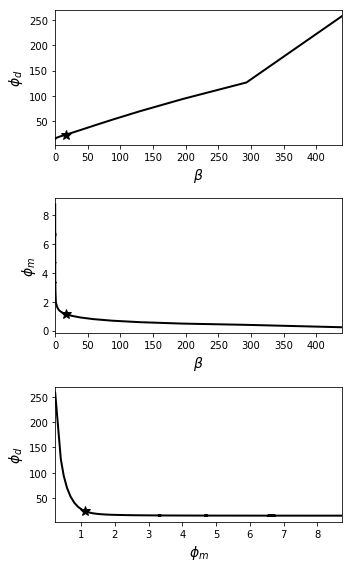In :
invProgress.plot_misfit_curves()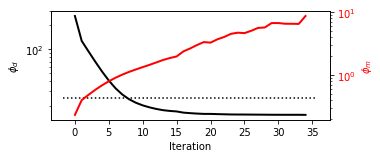## The results!¶

In what follows, we will generate an interactive plots that show

• (a) the true and recovered model

• (b) the Tikhonov curve with the target misfit plotted as a star, and the current iteration as a dot

• (c) predicted and observed apparent resistivity curve

• (d) predicted and observed phase curve

What does our data fit look like at each iteration? When have we underfit the data? overfit the data? Let's scroll through the inversion results and see.

In :
# grab beta, phi_d, phi_m from the inversion. They are lists, so we convert them to arrays
beta = np.array(invProgress.beta)
phi_d = np.array(invProgress.phi_d)
phi_m = np.array(invProgress.phi_m)

# find the iteration where we achieved target misfit
i_target = invProgress.i_target

print("The inversion reached target misfit after {} iterations".format(i_target))

The inversion reached target misfit after 8 iterations

In :
from ipywidgets import interact, IntSlider, ToggleButtons, fixed

In :
def view_1Dinversion_results(iteration, scale="linear", color="C0", alpha=1, ax=None, plotTrue=True):
if ax is None:
fig, ax = plt.subplots(2, 2, figsize=(12, 6))
ax = ax.flatten()

# get the apparent resistivity and phase data for this iteration
dpred = survey.dpred(xc[iteration])
app_res_pred, phase_pred = appres_phase_from_data(
dpred, frequency
)

# plot the true and recovered models
m_iter = xc[iteration]
sigtrue = np.repeat(sigma, 2, axis=0)
z = np.repeat(mesh.vectorCCx[1:], 2, axis=0)
z = np.r_[mesh.vectorCCx, z, mesh.vectorCCx[-1]]

if plotTrue is True:
ax.loglog(-z, sigtrue, 'k', lw=1, label='true')
ax.loglog(phi_m, phi_d, 'k-', lw=2)
ax.loglog(phi_m[i_target], phi_d[i_target], 'C3*', ms=16, label="target")
ax.loglog(frequency, app_res_obs, '.k-', linewidth=1, label='obs')
ax.semilogx(frequency, phase_obs, '.k-', linewidth=1, label="obs")

ax.loglog(-mesh.vectorCCx, np.exp(m_iter), color=color, alpha=alpha, lw=2, label='rec it {}'.format(iteration))
ax.set_ylabel("Conductivity (S/m)", fontsize = 14)
ax.set_xlabel("Depth (m)", fontsize = 14)
ax.set_ylim(2e-3, 2e-1)
ax.set_xlim((-z).min(), (-z).max())
ax.legend(loc=2)

# plot the Tikhonov curve
ax.loglog(phi_m[iteration], phi_d[iteration], color+'o', ms=8, label="it {}".format(iteration))
ax.set_xlim(phi_m.min(), phi_m.max())
ax.set_xlabel("$\phi_m$", fontsize = 14)
ax.set_ylabel("$\phi_d$", fontsize = 14)
ax.set_xscale(scale)
ax.legend()

# add iteration and beta values
ax.text(
phi_m[iteration], phi_d[iteration]*1.25,
"$\\beta$ = {:1.1e}".format(
beta[iteration]
), fontsize=11,  #, xy=(0, 0), xytext=(0, 0)
)

# plot the apparend resistivity data
ax.loglog(frequency, app_res_pred, color, alpha=alpha, label='pred, it {}'.format(iteration))
ax.set_xlim(frequency.max(), frequency.min())
ax.set_ylim(10, 3e2)
ax.set_xlabel("Frequency (Hz)", fontsize = 14)
ax.set_ylabel("$\\rho_a \ (\Omega m)$", fontsize = 14)
ax.legend(loc=1)

# plot the phase
ax.semilogx(frequency, phase_pred, color, alpha=alpha, label="pred, it {}".format(iteration))
ax.set_xlim(frequency.max(), frequency.min())
ax.set_xlabel("Frequency (Hz)", fontsize = 14)
ax.set_ylabel("Phase (degree)", fontsize = 14)
ax.legend(loc=1)

for a, title in zip(ax, ['(a)', '(c)', '(b)', '(d)']):
a.set_title(title, fontsize=14)
if title != "c":
a.grid(True, which='both', linewidth=0.4)

In :
# for ipywidets, all kwarg inputs must be specified, and plt.show() needs to be used
def interact_view_inversion(iteration, scale):
view_1Dinversion_results(iteration, scale)
plt.tight_layout()
plt.show()

interact(
interact_view_inversion,
iteration=IntSlider(min=0, max=beta.shape-1, step=1, value=0),
scale=ToggleButtons(options=["linear", "log"], value="log"),
)

Out:
<function __main__.interact_view_inversion>

Here is the figure from the article.

In :
fig, ax = plt.subplots(2, 2, figsize=(12, 6), dpi=400)
ax = ax.flatten()

for it, col, alpha, plotTrue in zip(
[5, 9, 21], [ "C1", "C0", "C2"], [0.5, 1., 0.5], [True, False, False]
):
view_1Dinversion_results(
iteration=it, scale="log", color=col, alpha=alpha, ax=ax, plotTrue=plotTrue
)

plt.tight_layout()